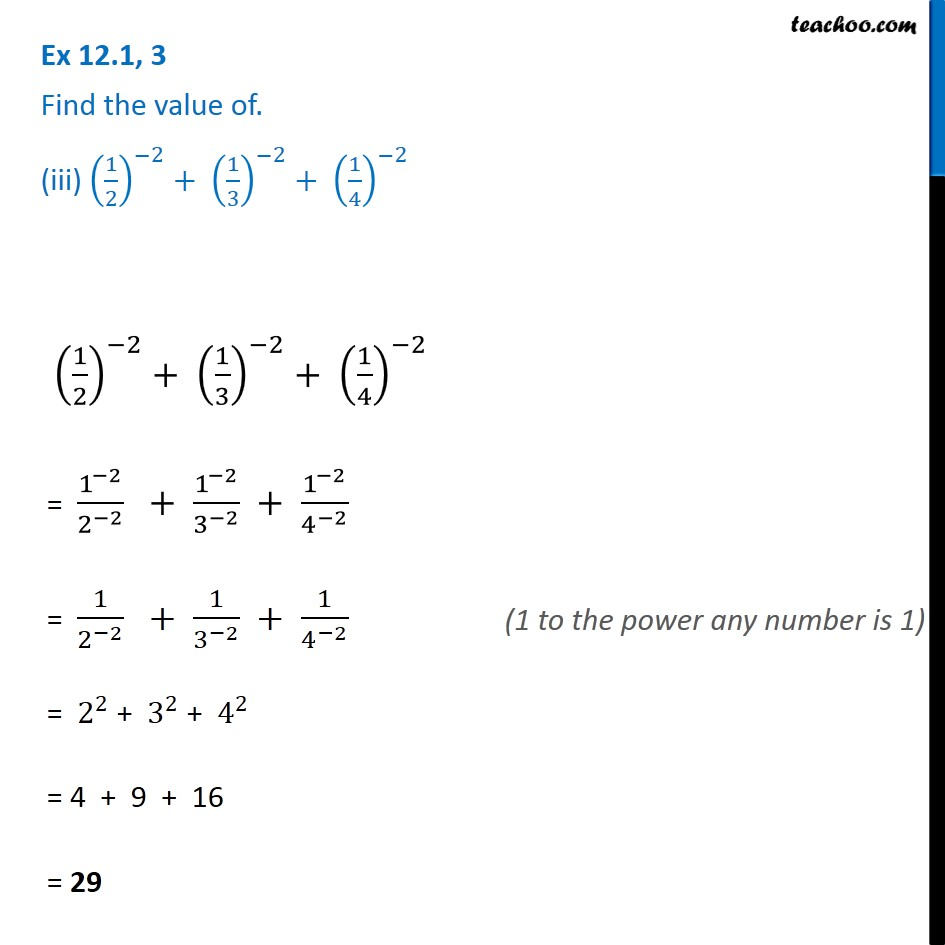Ex 12.1

Chapter 12 Class 8 Exponents and Powers
Serial order wiseGet live Maths 1-on-1 Classs - Class 6 to 12

### Transcript

Ex 12.1, 3 Find the value of. (iii) (1/2)^(−2)+ (1/3)^(−2)+ (1/4)^(−2) (1/2)^(−2)+ (1/3)^(−2)+ (1/4)^(−2) = 1^(−2)/2^(−2) + 1^(−2)/3^(−2) + 1^(−2)/4^(−2) = 1/2^(−2) + 1/3^(−2) + 1/4^(−2) = 2^2 + 3^2 + 4^2 = 4 + 9 + 16 = 29 (1 to the power any number is 1)